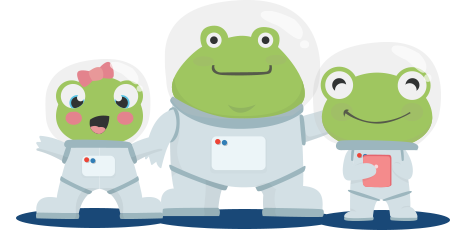# Year 3 Maths Printable Worksheets

Our fun and engaging maths worksheets cover all areas of the Y3 maths curriculum, including counting, mental maths, solving problems and calculations, shape and space, multiplication tables, number facts, measuring, data handling, and much more. Whether it's finding the perimeter of 2D shapes or using the 24-hour clock, you will be sure to find a year 3 maths worksheet to suit your child's needs.

Filters

### Mental maths 3

Mental maths is the process of doing mathematical calculations in your head. Students answer the questions as quickly as they can without the use of a calculator.

Age: 7 - 8
1
2
3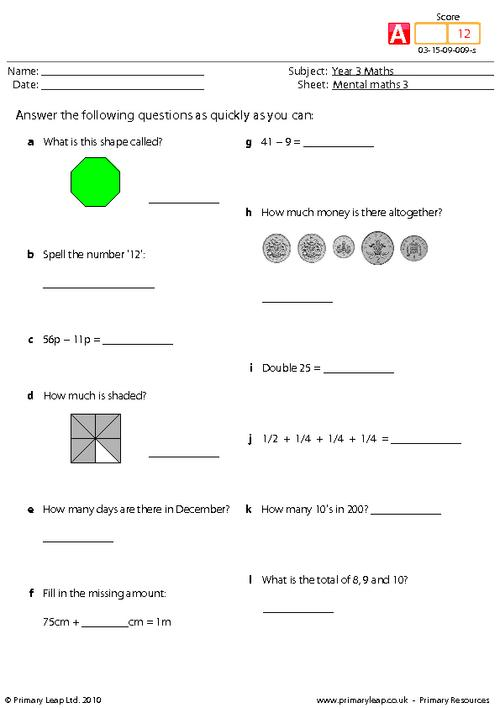### Mental maths 3

Mental maths is the process of doing mathematical calculations in your head. Students answer the questions as quickly as they can without the use of a calculator.

Age: 7 - 8
1
2
3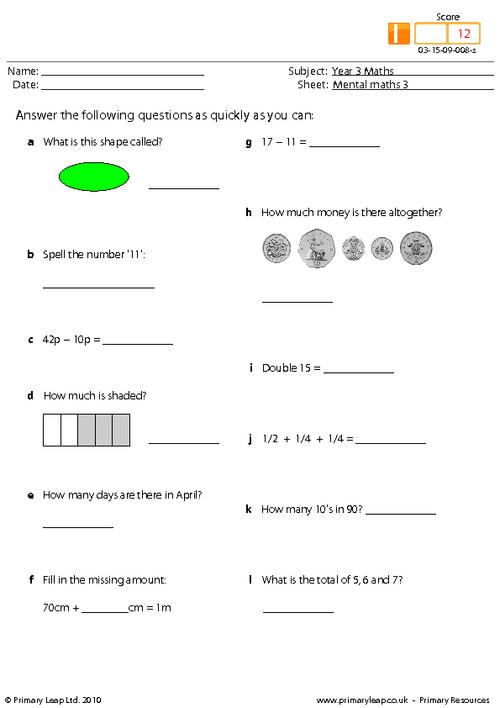### Mental maths 3

Mental maths is the process of doing mathematical calculations in your head. Students answer the questions as quickly as they can without the use of a calculator.

Age: 7 - 8
1
2
3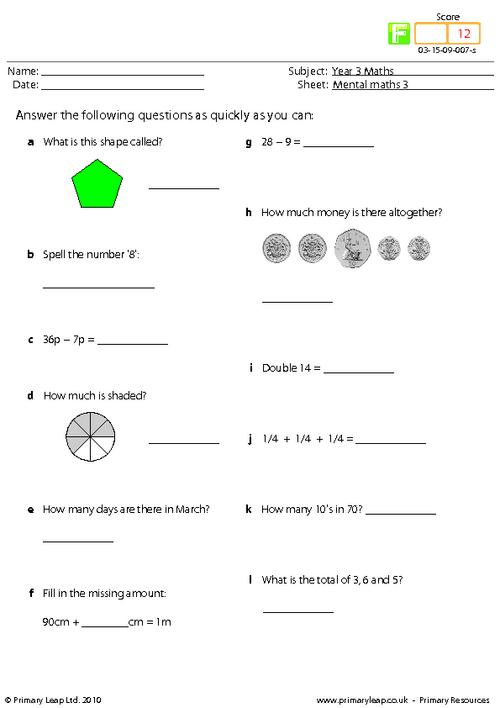### Mental maths 2

A year 3 mental maths worksheet. The child should answer all 12 questions as fast as they can to improve their mental skills.

Age: 7 - 8
1
2
3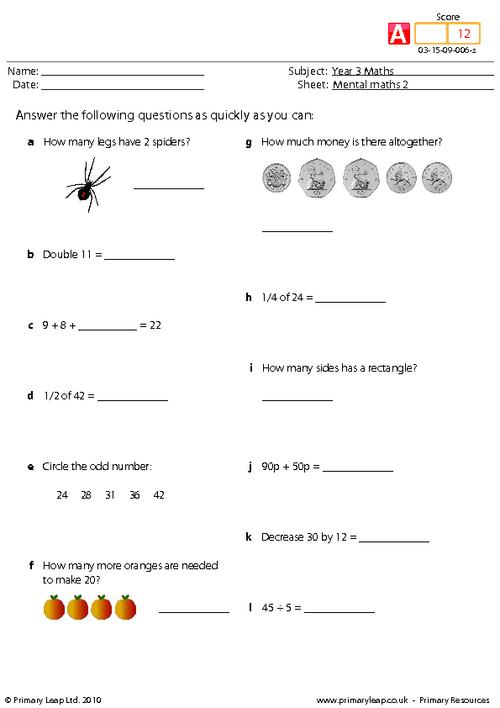### Mental maths 2

Mental maths is the process of doing mathematical calculations in your head. Students answer the questions as quickly as they can without the use of a calculator.

Age: 7 - 8
1
2
3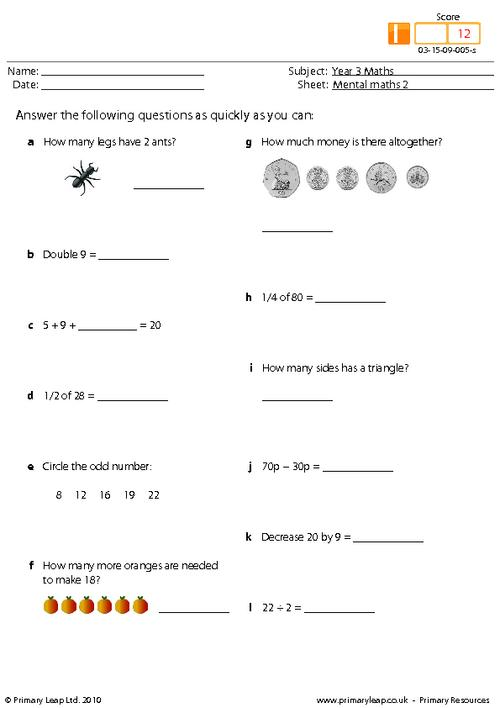### Mental maths 2

Mental maths is the process of doing mathematical calculations in your head. Students answer the questions as quickly as they can without the use of a calculator.

Age: 7 - 8
1
2
3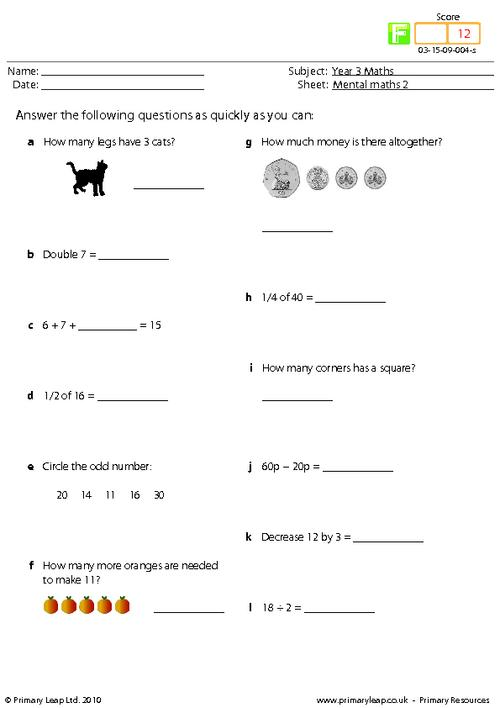### Mental maths 1

Mental maths is the process of doing mathematical calculations in your head. Students answer the questions as quickly as they can without the use of a calculator.

Age: 7 - 8
1
2
3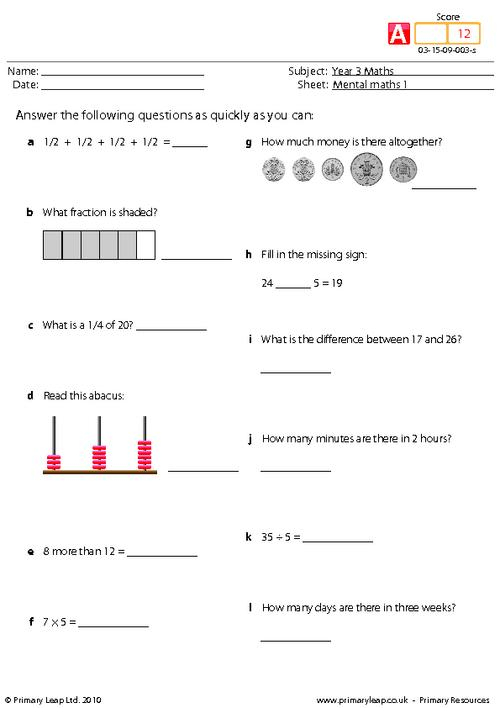### Mental maths 1

Mental maths is the process of doing mathematical calculations in your head. Students answer the questions as quickly as they can without the use of a calculator.

Age: 7 - 8
1
2
3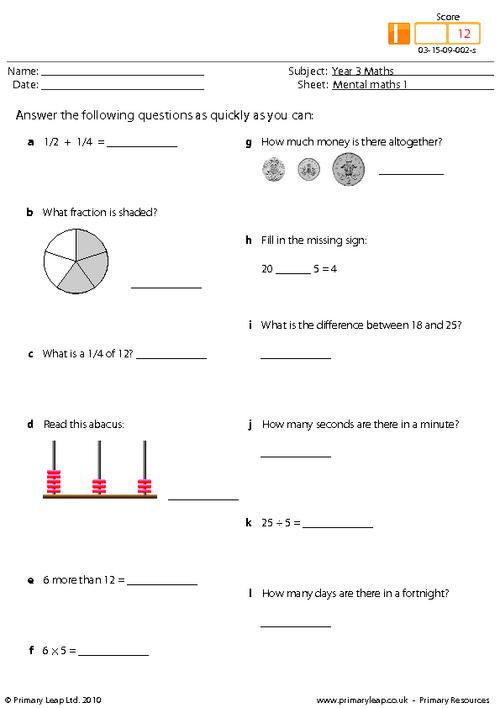### Mental maths 1

Mental maths is the process of doing mathematical calculations in your head. Students answer the questions as quickly as they can without the use of a calculator.

Age: 7 - 8
1
2
3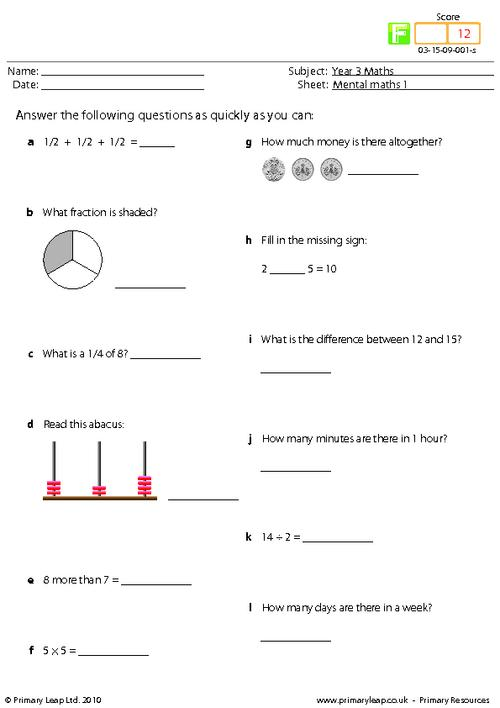### Mental Maths 6

A year 3 mental maths resource.

Age: 7 - 8
1
2
3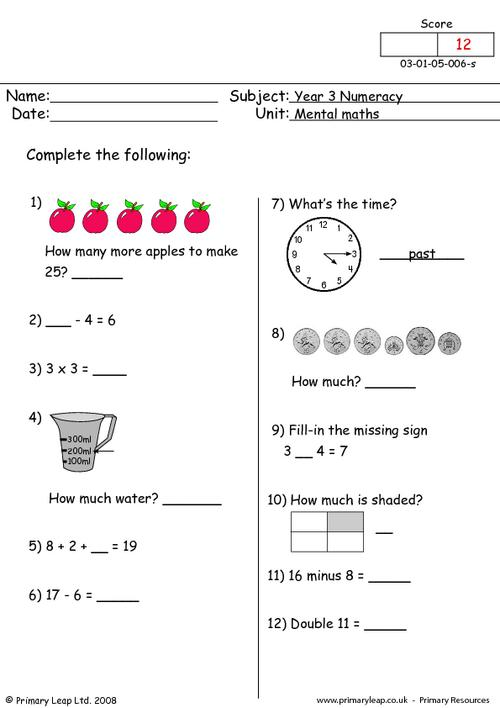### Mental Maths 5

A year 3 maths worksheet on mental maths.

Age: 7 - 8
1
2
3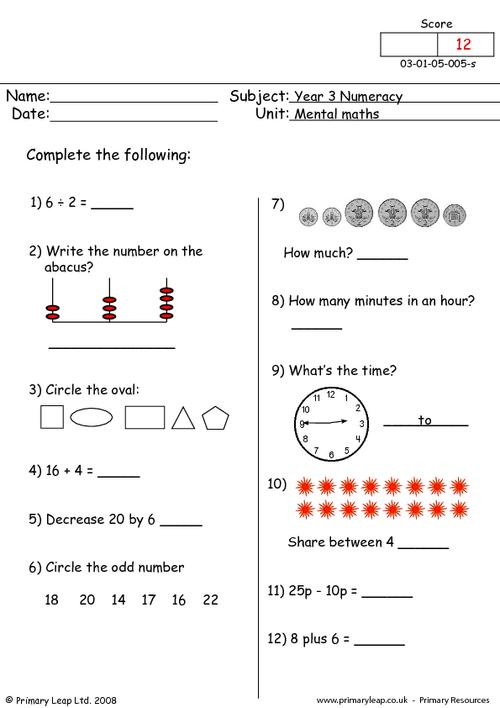### Mental Maths 3

A year 3 mental maths resource with 12 questions to answer.

Age: 7 - 8
1
2
3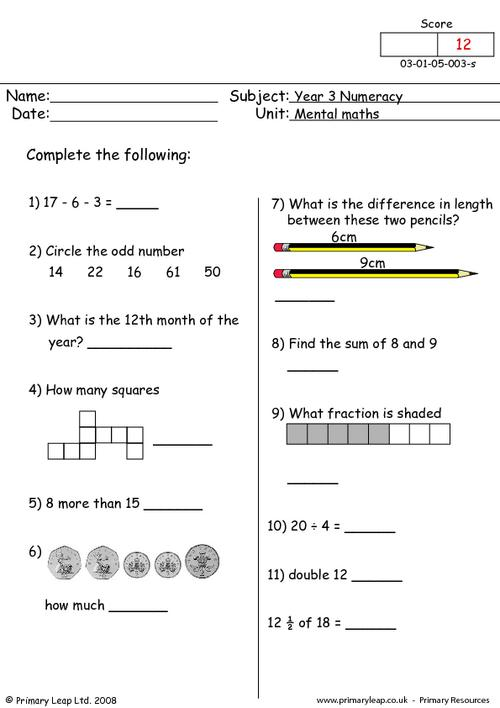### Mental Maths 2

A KS2 mental maths resource with 12 questions to answer.

Age: 7 - 8
1
2
3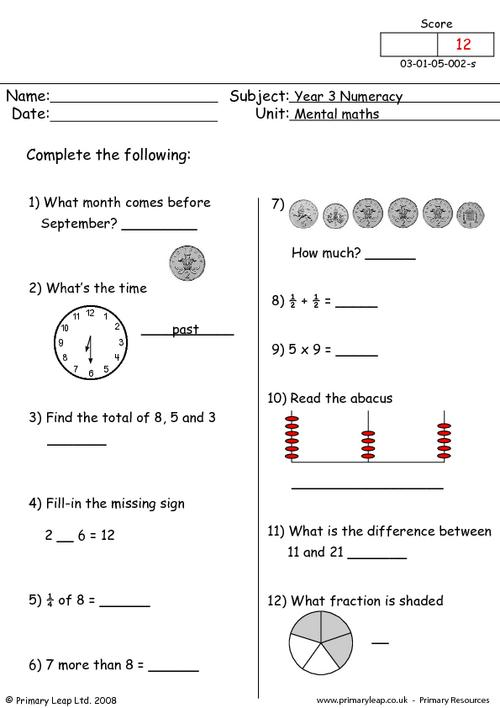### Mental Maths 1

This is a mental maths primary resource for year 3.

Age: 7 - 8
1
2
3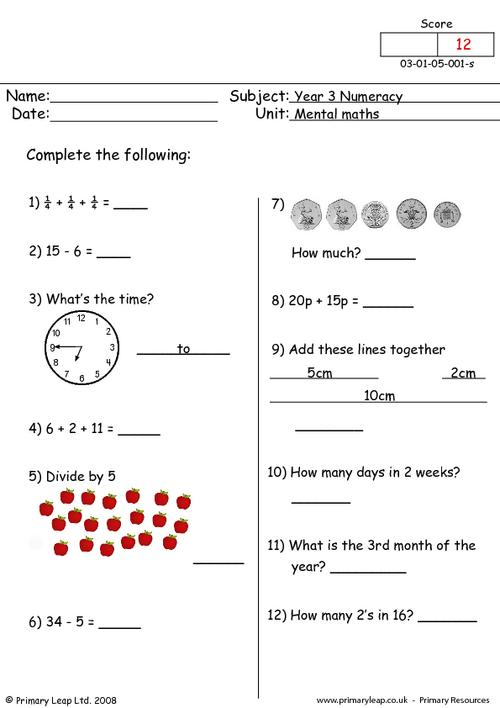### Mental maths 7

A KS2 maths resource on mental maths.

Age: 7 - 8
1
2
3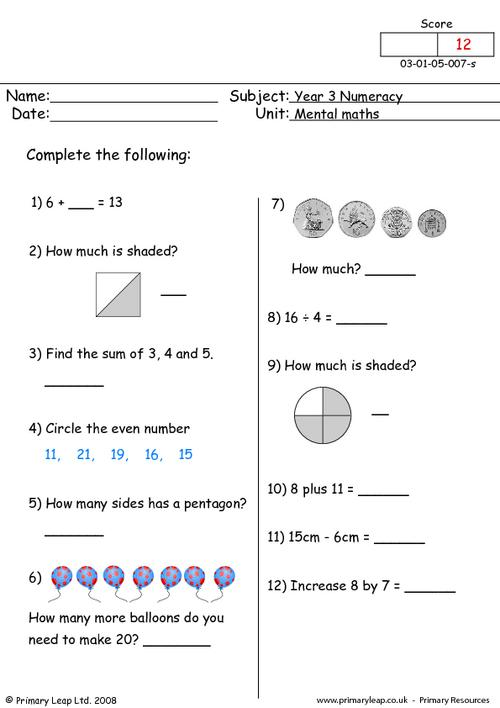of 1We have hundreds of year 3 maths worksheets to help your child feel more confident in their own abilities and continue to develop their understanding of numbers. Our maths worksheets will help your child learn more about fractions, including tenths, find perimeters of 2D shapes, recognise angles, use bar charts, and much more.Learn

One of the best things about printable worksheets is that they make learning fun and easy. Explore our educational resources based on Year 3 Maths and keep your children learning through these fun and engaging exercises.Progress

Our Year 3 Maths printable worksheets are designed for progressive learning. Our engaging content enables a child to learn at their own speed and gain confidence quickly. When we help a child believe in themselves, we empower them for life.Improve

Printable worksheets are a great way to enhance creativity and improve a child’s knowledge. Help your child learn more about Year 3 Maths with this great selection of worksheets designed to help children succeed.